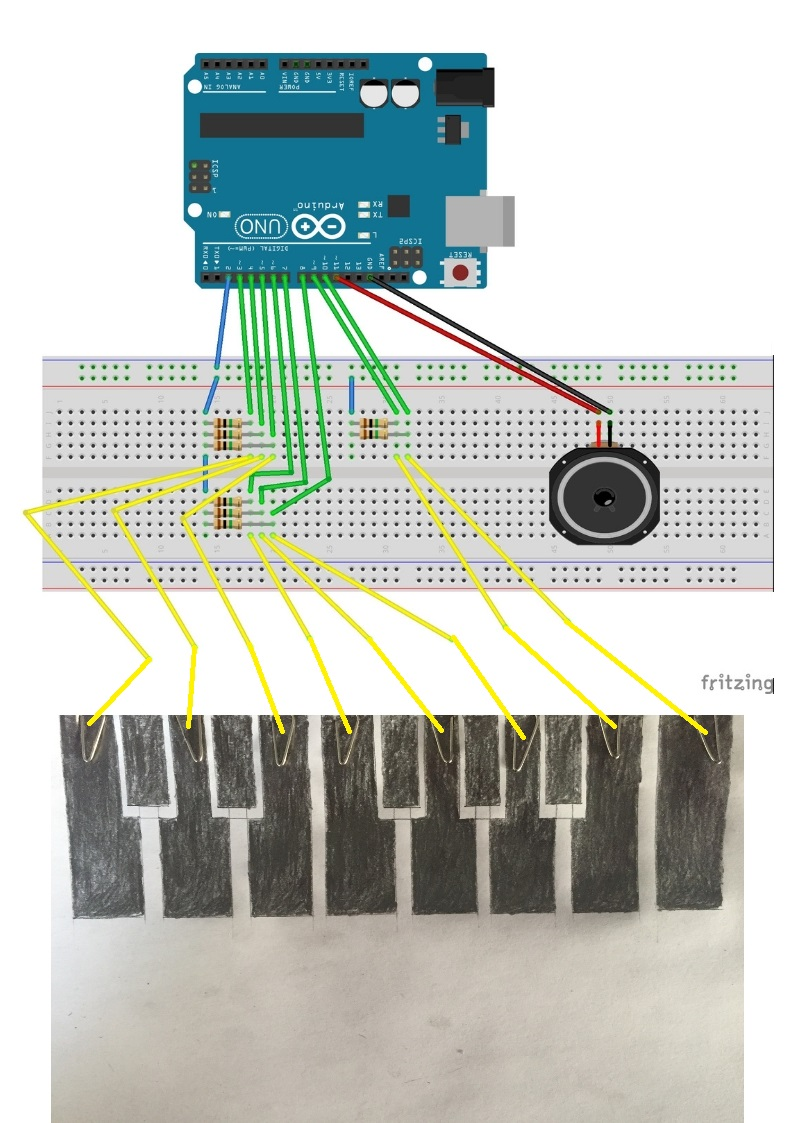Project showcase

# Paper Piano with Arduino © GPL3+

This project uses an Arduino, a drawn keyboard using lead pencil, a paper, and a speaker.

• 55,913 views
• 158 respects

## Components and supplies

 Male-to-male jumper wires
×1
×1
 Arduino
×1
 Resistor 1M ohm
×1
 Speaker
×1

This is a simple project using an Arduino, a drawn keyboard using lead pencil, a paper, and a speaker.

### Materials

- Male-to-male jumper wires : https://amzn.to/37kTL0M

- Arduino Uno board : https://amzn.to/39cqZ3E

- Resistor 1M ohm : https://amzn.to/2oY0SYJ

- Speaker : https://amzn.to/2PHBgNC

- Pencil

- A4 paper

- Paper clip

### Capacitive Sensing

Capacitive touch sensing is a way of human touch sensing, that requires little or no force to activate. It may be used to sense human touch through more than a quarter of an inch of plastic, wood, ceramic or other insulating material (not any kind of metal though), enabling the sensor to be completely visually concealed.

### Why Capacitive Touch?

• Each touch sensor requires only one wire connected to it.
• Can be concealed under any non-metallic mmaterial.
• Can be easily used in place of a button.
• Can detect a hand from a few inches away, if required.
• Very inexpensive.

### How Does a Capacitive Sensor Work?

The sensor plate and your body forms a capacitor. We know that a capacitor stores charge. The more its capacitance, the more charge it can store.

The capacitance of this capacitive touch sensor depends on how close your hand is to the plate.

### What Does the Arduino Do?

Basically the Arduino measures how much time the capacitor (i.e the touch sensor) takes to charge, giving it an estimate of the capacitance.

The capacitance may be very small, nevertheless the Arduino measures it with accuracy.

One way of using capacitive touch in a project is to use the CapSense library. For the CapSense library, the arduino uses one send pin and any number of receive pins required. A receive pin is connected to the send pin via a medium to high value resistor.

Here are some guidelines for resistors but be sure to experiment for a desired response.

• Use a 1 megohm resistor (or less maybe) for absolute touch to activate.
• With a 10 megohm resistor the sensor will start to respond 4-6 inches away.

## Code

##### Paper_piano.inoArduino
```/* Original project by Oh Hai Seng 07 November 2013
To watch the Demo of his Project:

*/

// Import the CapacitiveSensor Library.
#include <CapacitiveSensor.h>

#define speaker 11

// Set the Send Pin & Receive Pin.
CapacitiveSensor   cs_2_3 = CapacitiveSensor(2,3);
CapacitiveSensor   cs_2_4 = CapacitiveSensor(2,4);
CapacitiveSensor   cs_2_5 = CapacitiveSensor(2,5);
CapacitiveSensor   cs_2_6 = CapacitiveSensor(2,6);
CapacitiveSensor   cs_2_7 = CapacitiveSensor(2,7);
CapacitiveSensor   cs_2_8 = CapacitiveSensor(2,8);
CapacitiveSensor   cs_2_9 = CapacitiveSensor(2,9);
CapacitiveSensor   cs_2_10 = CapacitiveSensor(2,10);

void setup()
{
cs_2_6.set_CS_AutocaL_Millis(0xFFFFFFFF);     // turn off autocalibrate on channel 1 - just as an example

// Arduino start communicate with computer.
Serial.begin(9600);
}

void loop()
{
// Set a timer.
long start = millis();

// Set the sensitivity of the sensors.
long total1 =  cs_2_3.capacitiveSensor(3000);
long total2 =  cs_2_4.capacitiveSensor(3000);
long total3 =  cs_2_5.capacitiveSensor(3000);
long total4 =  cs_2_6.capacitiveSensor(3000);
long total5 =  cs_2_7.capacitiveSensor(3000);
long total6 =  cs_2_8.capacitiveSensor(3000);
long total7 =  cs_2_9.capacitiveSensor(3000);
long total8 =  cs_2_10.capacitiveSensor(3000);

Serial.print(millis() - start);        // check on performance in milliseconds
Serial.print("\t");                    // tab character for debug windown spacing

Serial.print(total1);                  // print sensor output 1
Serial.print("\t");                    // Leave some space before print the next output
Serial.print(total2);                  // print sensor output 2
Serial.print("\t");                    // Leave some space before print the next output
Serial.print(total3);                  // print sensor output 3
Serial.print("\t");                    // Leave some space before print the next output
Serial.print(total4);                  // print sensor output 4
Serial.print("\t");                    // Leave some space before print the next output
Serial.print(total5);                  // print sensor output 5
Serial.print("\t");                    // Leave some space before print the next output
Serial.print(total6);                  // print sensor output 6
Serial.print("\t");                    // Leave some space before print the next output
Serial.print(total7);                   // print sensor output 7
// Leave some space before print the next output
Serial.print("\t");
Serial.println(total8);                 // print sensor output 8
// "println" - "ln" represent as "line", system will jump to next line after print the output.

// When hand is touched the sensor, the speaker will produce a tone.
// I set a threshold for it, so that the sensor won't be too sensitive.
if (total1 > 500) tone(speaker,131);   // frequency
if (total2 > 500) tone(speaker,147);   // you can see https://www.arduino.cc/en/Tutorial/toneMelody if you want to change frequency
if (total3 > 500) tone(speaker,165);
if (total4 > 500) tone(speaker,175);
if (total5 > 500) tone(speaker,196);
if (total6 > 500) tone(speaker,220);
if (total7 > 500) tone(speaker,247);
if (total8 > 500) tone(speaker,262);

// When hand didn't touch on it, no tone is produced.
if (total1<=500  &  total2<=500  &  total3<=500 & total4<=500  &  total5<=500  &  total6<=500 &  total7<=500 &  total8<=500)
noTone(speaker);

delay(10);                             // arbitrary delay to limit data to serial port
}
```

## Schematics#### Electronic Piano Keyboard With Preset Songs

Project tutorial by Lindsay Fox

• 108,075 views
• 231 respects

#### Touch Sensing Paper Piano

Project tutorial by Jeremy Sow

• 3,447 views
• 1 comment
• 10 respects

#### Unravel Preset Piano Easy Arduino (Even a Ghoul Can Make It)

Project in progress by ExeCuteLi

• 27,678 views
• 50 respects

• 17,008 views
• 77 respects

#### Arduino Tutorial : Mini Piano

Project tutorial by Rahul Khanna D

• 43,047 views